# Vertical Line

In a coordinate plane, a line parallel to the Y-axis is called Vertical Line. It is a straight line which goes from top to bottom and bottom to top. Any point in this line will have the same value for the x-coordinate. For example, (2,0), (3,0) (-4,0), etc. are the points of vertical lines. Similarly, the line which goes from left to right and is parallel to the x-axis is called a horizontal line.

The vertical lines have no slope. It goes parallelly along with y-axis and hence the slope is not defined. Therefore, the equation of the vertical line which crosses the x-axis at any point say ‘a’, is given by:

x = a

where x is the coordinate of a point on the line and ‘a’ is the point where the lines cross the x-intercept. See the below image to understand the difference between vertical and horizontal lines.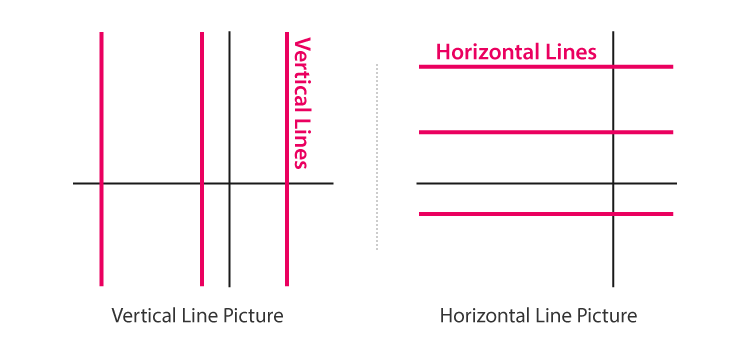In the above figure, we can see, the left side shows the vertical lines in a coordinate plane whereas the right side shows the horizontal lines parallel to the x-axis.

## Properties of Vertical Lines

• The equation of a vertical line in the graph, which is parallel to y-axis is x = a.
• The slope of a vertical line is infinity or undefined as it has no y-intercept and the denominator in the slope formula is zero.
• To check whether the relation is a function in maths, we use a vertical line. It would be a function if all vertical lines intersect it minimum once. This is also called a vertical line test. A graph will be considered as a function if it has only one output y for each input x. Therefore, a vertical line cannot be a function.

## Solved Examples

Question 1: Plot the graph for the following:

1. X = 8

x=8 is a vertical line, that passes through x-axis, at a distance of 8 units on the right of the origin and is parallel to y-axis.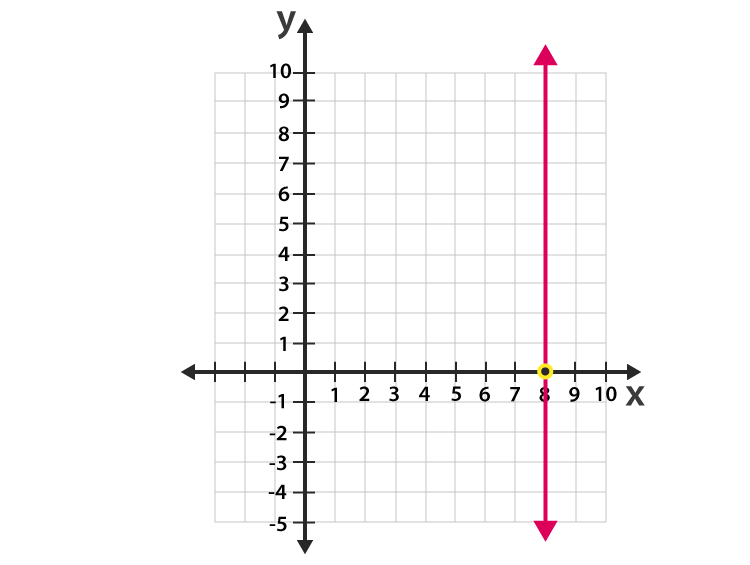1. X = – 4

x=-4 is a vertical line, that passes through x-axis, at a distance of 4 units on the left side of the origin and is parallel to y-axis.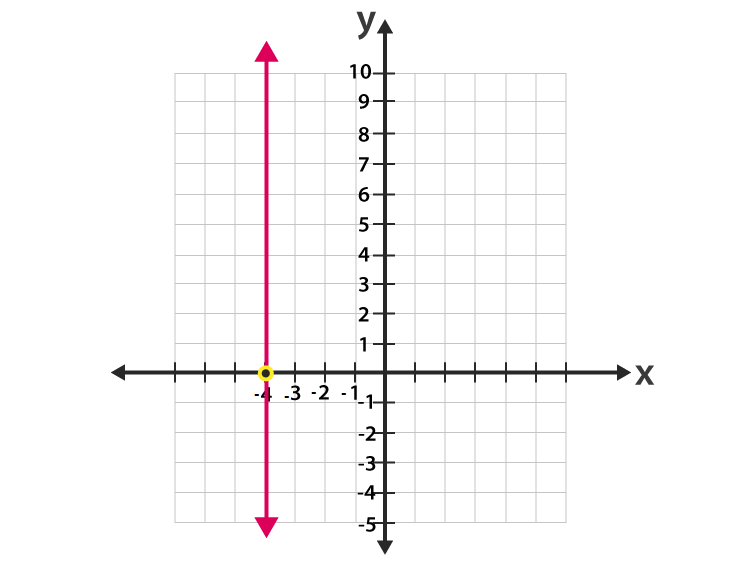1. X = 3

x=3 is a vertical line, that passes through x-axis, at a distance of 3 units on the right side of the origin and is parallel to y-axis.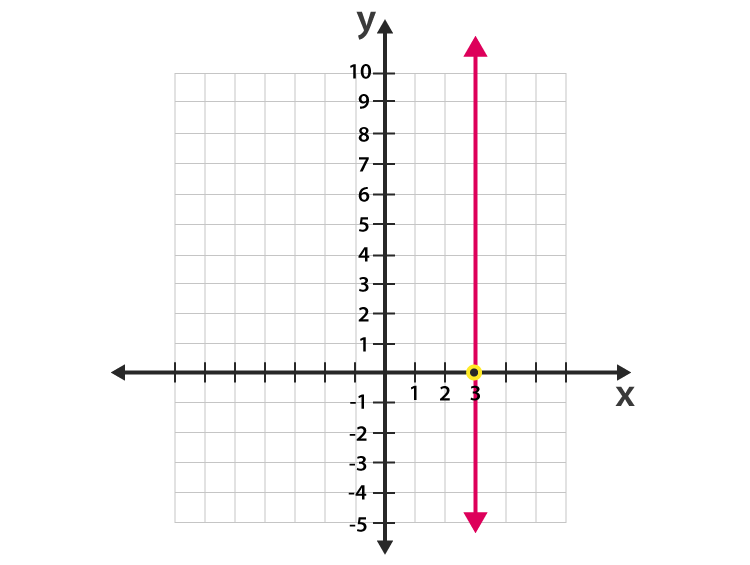1. X = – 5

x=-5 is a vertical line, that passes through x-axis, at a distance of 5 units on the left of the origin and is parallel to y-axis.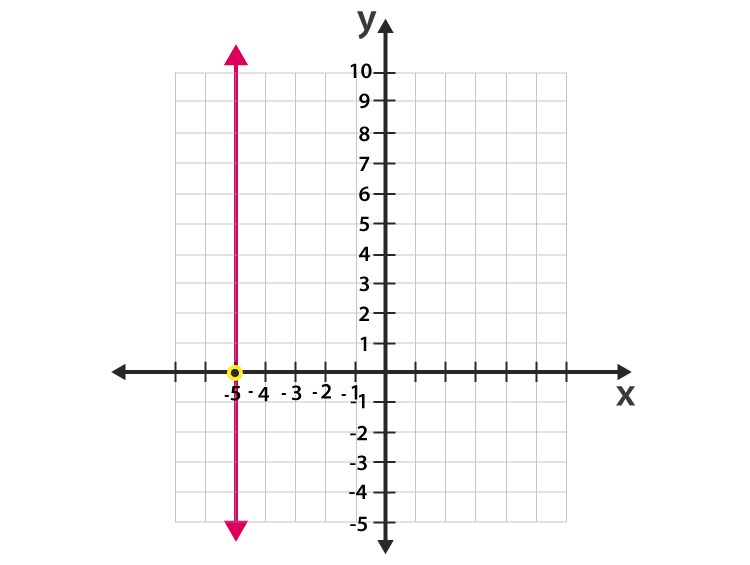1. X = 6

x=6 is a vertical line, that passes through x-axis, at a distance of 6 units on the right side of the origin and is parallel to y-axis.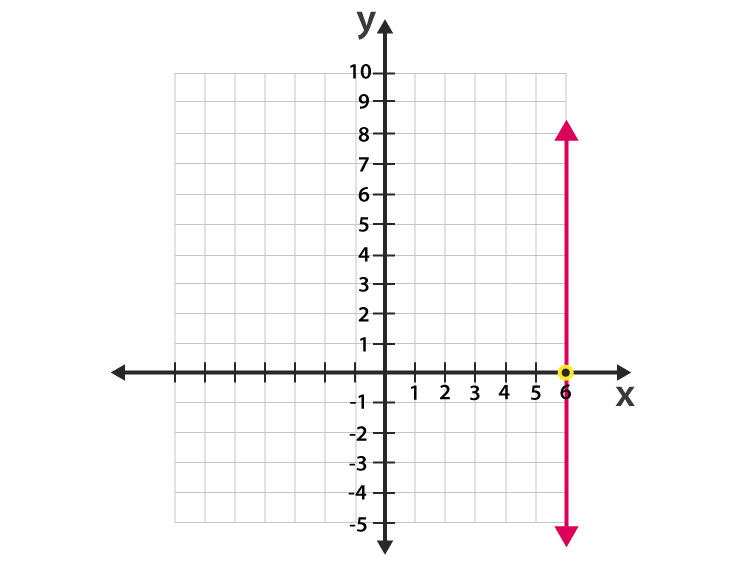## Frequently Asked Questions – FAQs

Q1

### What is vertical and horizontal line?

A vertical line is a line, parallel to y-axis and goes straight, up and down, in a coordinate plane. Whereas the horizontal line is parallel to x-axis and goes straight, left and right.
Q2

### What is the equation of vertical line?

The equation of vertical line is given by: x = a
where x is the x-coordinate of any point on line and a is the crossing point of vertical line on x-axis.
Q3

### What way does the vertical line go?

A vertical line goes straight from top to bottom and bottom to top in a coordinate plane.
Q4

### Is x=0, a vertical line?

x=0 is a vertical line that passes through the origin and is parallel to y-axis.
Q5

### Is x = -3, a vertical line?

x=-3 is a vertical line, that passes through x-axis, at a distance of 3 units on the left of the origin and is parallel to y-axis.